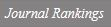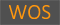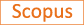NOTE ON SOME OPERATOR EQUATIONS AND LOCAL SPECTRAL PROPERTIES

Title
NOTE ON SOME OPERATOR EQUATIONS AND LOCAL SPECTRAL PROPERTIES
Authors
An, Il JuKo, Eungil
Ewha Authors
SCOPUS Author ID
고응일Issue Date
2016
Journal Title
OPERATORS AND MATRICES
ISSN
1846-3886Citation
OPERATORS AND MATRICES vol. 10, no. 2, pp. 397 - 417
Keywords
Operator equationsspectrumsingle valued extension property
Publisher
ELEMENT
Indexed
SCIE; SCOPUSDocument Type
Article
Abstract
In this paper we define J(k, j) by the set of solutions (A, B) of the operator equations A(k)B(j+1)A(k) = A(2k+j) and B(k)A(j+1)B(k) = B2k+j. Then we observe the set J(k,j) is increasing for all integers k >= 1 and j >= 0. Now let a pair (A, B) is an element of J(k,j) boolean AND J(j+1,k-1) for any integer k >= 1 and j >= 0. We show that if any one of the operators A, AB, BA, and B has Bishop's property (beta), then all others have the same property. Furthermore, we prove that the operators A(k+j), A(k)B(j+1), A(j+1)B(k), B(j+1)A(k), B(k)A(j+1) and Bk+j have the same spectra and spectral properties. Finally, we investigate their Weyl type theorems.
DOI
10.7153/oam-10-22
Appears in Collections:
자연과학대학 > 수학전공 > Journal papers
Files in This Item:
There are no files associated with this item.
Export
RIS (EndNote)
XLS (Excel)
XML